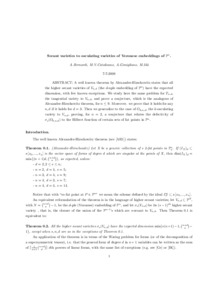# Secant varieties to osculating varieties of Veronese embeddings of $P^n$.

Bernardi, Alessandra ; Catalisano, Maria Virginia ; Gimigliano, Alessandro ; Idà, Monica (2008) Secant varieties to osculating varieties of Veronese embeddings of $P^n$. [Preprint]
Full text available as:Preview PDF Download (229kB) | Preview

## Abstract

A well known theorem by Alexander-Hirschowitz states that all the higher secant varieties of $V_{n,d}$ (the $d$-uple embedding of $\PP n$) have the expected dimension, with few known exceptions. We study here the same problem for $T_{n,d}$, the tangential variety to $V_{n,d}$, and prove a conjecture, which is the analogous of Alexander-Hirschowitz theorem, for $n\leq 9$. Moreover. we prove that it holds for any $n,d$ if it holds for $d=3$. Then we generalize to the case of $O_{k,n,d}$, the $k$-osculating variety to $V_{n,d}$, proving, for $n=2$, a conjecture that relates the defectivity of $\sigma_s(O_{k,n,d})$ to the Hilbert function of certain sets of fat points in $\PP n$.

Abstract
Document type
Preprint
Creators
CreatorsAffiliationORCID
Bernardi, Alessandra
Catalisano, Maria Virginia
Gimigliano, Alessandro
Idà, Monica
Keywords
Varietà delle Secanti, Varietà di Veronese, Varietà Osculanti, Varietà Tangenziali, Lemma d'Horace Differenziale
Subjects
DOI
Deposit date
15 Jul 2008